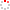#CodeStepByStep## switchPairsOfPairs

Write a function named `switchPairsOfPairs` that rearranges a linked list of integers by switching the order of each two neighboring pairs of elements in the sequence. Your function is passed a reference to a pointer to the front of the list as a parameter. Suppose, for example, that a variable named `list` points to the front of a list storing the following values:

```{1, 2, 3, 4, 5, 6, 7, 8}
|  |  |  |  |  |  |  |
+--+  +--+  +--+  +--+
pair  pair  pair  pair
```

This list has four pairs. If we make the call of `switchPairsOfPairs(front);` the list's state should become:

```{3, 4, 1, 2, 7, 8, 5, 6}
|  |  |  |  |  |  |  |
+--+  +--+  +--+  +--+
pair  pair  pair  pair

```

Notice that the pair (1, 2) has been switched with the pair (3, 4) and that the pair (5, 6) has been switched with the pair (7, 8). This example purposely used sequential integers to make the rearrangement clear, but you should not expect that the list will store sequential integers. It also might have extra values at the end that are not part of a group of four. Such values should not be moved. For example, if the list had stored this sequence of values:

```{3, 8, 19, 42, 7, 26, 19, -8, 193, 204, 6, -4, 99, 2, 7}
```

Then a call on the function should modify the list to store the following. Note that 99, 2, 7 at the end are not switched:

```{19, 42, 3, 8, 19, -8, 7, 26, 6, -4, 193, 204, 99, 2, 7}
```

Your function should work properly for a list of any size. Note: The goal of this problem is to modify the list by modifying pointers. It might be easier to solve it in other ways, such as by changing nodes' `data` values or by rebuilding an entirely new list, but such tactics are forbidden.

Constraints:

• Your code should run in no worse than O(N) time, where N is the length of the list. Your code must make a single pass over the list (not multiple passes).
• Note that the list has no "size" function, nor are you allowed to loop over the whole list to count its size.
• Do not use any auxiliary data structures such as arrays, vectors, queues, strings, maps, sets, etc.
• Do not modify the `data` field of any nodes; you must solve the problem by changing the links between nodes.
• You may not construct new `ListNode` objects, though you may create as many `ListNode*` pointers as you like.

Assume that you are using the `ListNode` structure as defined below:

```struct ListNode {
int data;         // value stored in each node
ListNode* next;   // pointer to next node in list (nullptr if none)
};
```
Type your C++ solution code here:

This is a function exercise. Write a C++ function as described. Do not write a complete program; just the function(s) above.